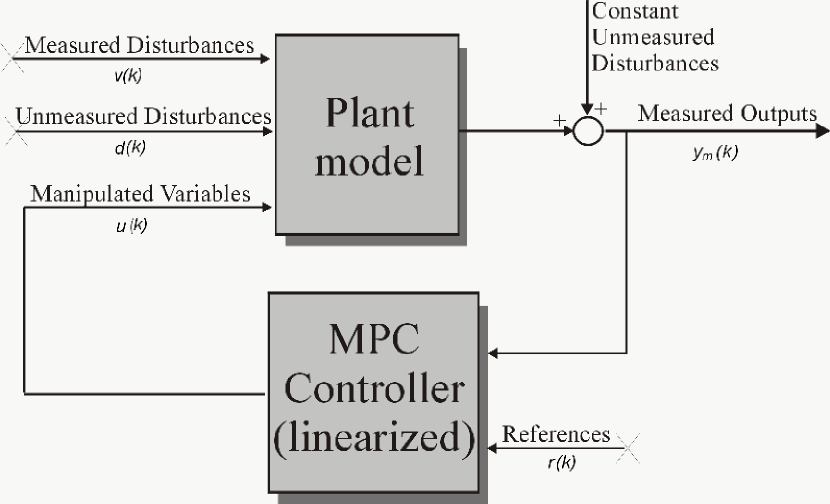# cloffset

Compute MPC closed-loop DC gain from output disturbances to measured outputs assuming constraints are inactive at steady state

## Syntax

```DCgain = cloffset(MPCobj)
```

## Description

The `cloff` function computes the DC gain from output disturbances to measured outputs, assuming constraints are not active, based on the feedback connection between `Model.Plant` and the linearized MPC controller, as depicted below.

Computing the Effect of Output DisturbancesBy superposition of effects, the gain is computed by zeroing references, measured disturbances, and unmeasured input disturbances.

`DCgain = cloffset(MPCobj)` returns an nym-by-nym DC gain matrix `DCgain`, where nym is the number of measured plant outputs. `MPCobj` is the MPC object specifying the controller for which the closed-loop gain is calculated. `DCgain(i,j)` represents the gain from an additive (constant) disturbance on output `j` to measured output `i`. If row `i` contains all zeros, there will be no steady-state offset on output `i`.